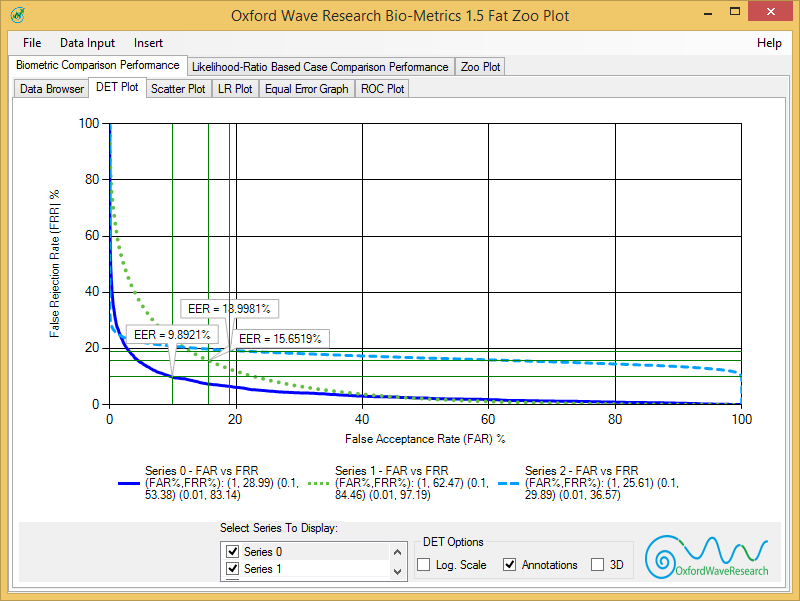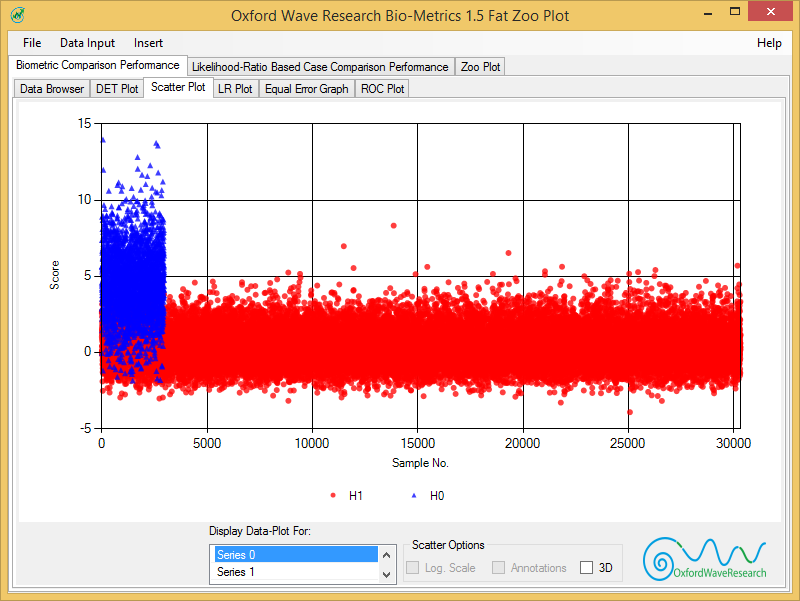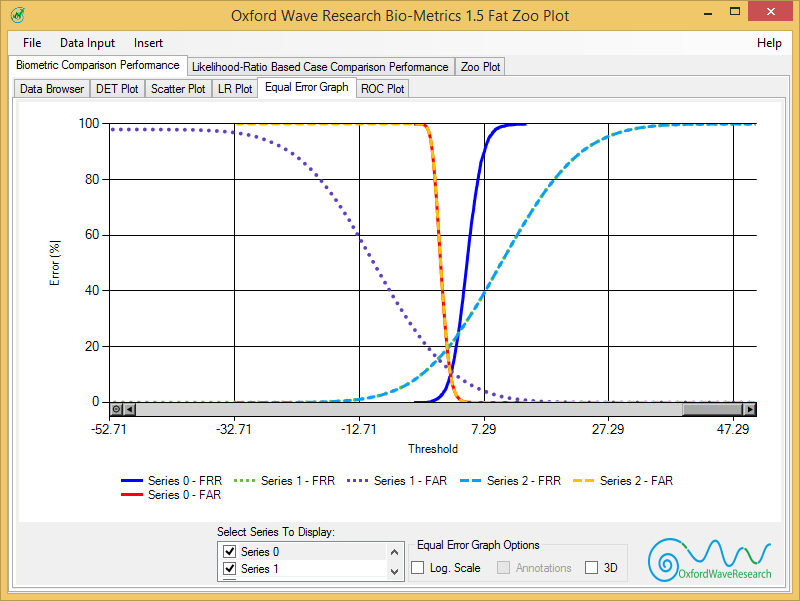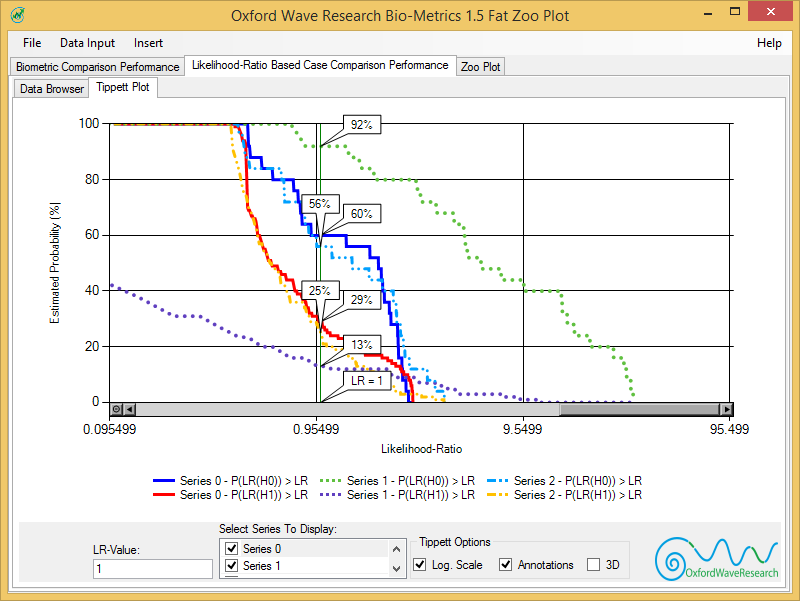# Bio-Metrics# Bio-Metrics – Performance Metrics Software

Calculating and plotting performance metrics for speaker and biometric recognition made easy.

Oxford Wave Research presents the Bio-Metrics software for the Windows platform that makes evaluating the performance of your speaker, biometric recognition systems, or algorithms easy and straightforward.

• Have you spent hours trying to calculate and plot DET plots, Tippett plots, or equal error graphs for your reports and scientific papers?
• Would you like a graphical and interactive way of calculating likelihood ratios (LRs) and calculating error metrics?
• Would you like to use graphical tools that are available for the Windows platform to make calculations easier?
• Would you like to easily export your results to Microsoft Word, or Powerpoint?

Our Bio-Metrics software provides you with the capability to quickly plot state of the art performance metrics for speaker recognition or other biometric recognition software. In order to do so, it requires the set of similarity scores obtained from comparing features of the same individual as well the set of scores obtained from comparing features of different individuals.

### Features

Data-browser: Allows you to observe and verify the values loaded for plotting. It is possible to also make minor changes of the data which are immediately reflected in the graphing.

Detection Error Trade-off (DET) plot: Helps to indicate the performance of the system by plotting false match rate against the false non-match rate for a range of score thresholds. This will also estimate the widely used equal error rate (EER) which is the point at which the false match rate is equal to the false non-match rate. The axes of the DET plot are generally plotted with logarithmic scales, although linear scales can also be equally informative.

Scatter-plot: Provides a quick overview of the data-points that are being plotted. This will help you to spot any erroneous data-points easily and also to observe general trends of the data.

Likelihood Ratio (LR) plot: Estimates the probability density functions (PDF) pertaining to the same-speaker comparisons and different speaker comparisons. You can then select a given point on this graph to calculate the likelihood ratio of that score belonging to a same-speaker comparison or a different speaker comparison. Please note that if scores obtained comparing the speaker’s voice with himself are provided, then this can be used to calculate the likelihood ratio explicitly calculating the between sources variability and the within sources variability.

Equal Error Graph: Plots the false acceptance rate or false match rate, and the false rejection rate or the false non-match rate on the vertical axis against the threshold score on the horizontal axis. The point of intersection of these two curves is the equal error rate, i.e. when the false acceptance rate is equal to the false rejection rate.

Receiver Operating Characteristic (ROC) Plot: Plots the false acceptance rate or false match rate (with respect to a threshold score) on the horizontal axis, with the correct acceptance rate plotted on the vertical. In other words, the receive operator curve plots the false match rate against the ‘true’ match rate, i.e. correctly accepting a genuine match. The true match rate is the likelihood of correctly accepting a genuine match, or 100-FAR. The x-axis of an ROC graph is typically logarithmic.

Tippett plots: A cumulative probability distribution plot that represents the proportion of the likelihood ratios greater than a given LR, i.e., P(LR(Hi) > LR), for cases corresponding to the hypotheses H0 and H1 true. The separation between the two curves in this representation is an indication of the performance of the system or method, with a larger separation implying better performance than a smaller one.

### Interactive Graphing Features

Zoom: Highlight a section of the chart, and the S-Metrics will then zoom and display that section. It is possible to get better resolution of any area of the graph of interest by doing this

Data-cursor: Hovering the mouse over the curves in the graph will display the horizontal and vertical axis values of the data at that point

Logarithmic Scale: For some of the graphs, it is possible to shift between a linear and a logarithmic (base 10) display of the values. This is useful in order to get better resolution at the lower values

Annotations: It is possible to turn the annotations (such as the likelihood ratio values, equal error rates, etc.) on and off

3D: The graph can be displayed in 3D which is useful for visualization as well as use in presentations and reports

### Supported Output Formats

Bio-Metrics allows the user to export the resulting graphs in either vector or raster image formats. The following output formats are supported.

• EMF: Enhanced Metafile (Vector format) supported by Microsoft Windows
• PNG: Portable Network Graphic (Raster format)
• JPEG: (Raster format)
• BMP: (Windows bitmap)

These formats allow for easy export into word documents and PowerPoint presentationsScatter PlotEqual Error GraphTippett Plot